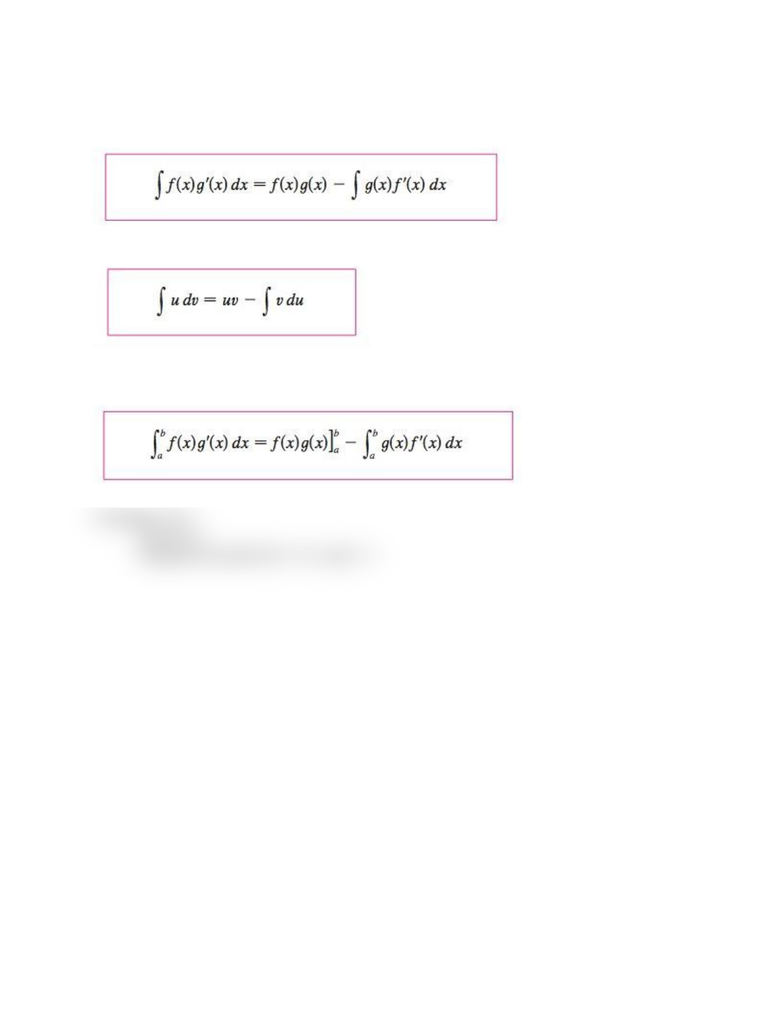Textbook Notes (280,000)
US (110,000)
UCSB (700)
MATH 3B (10)
helms (10)
Chapter 7.1

# Math 3B_ Chapter 7.1 Integration by Parts.docx

This preview shows half of the first page. to view the full 1 pages of the document.Chapter 7.1 Integration by Parts
formula for integration by parts
easier to remember notation
Combine formula for integration by parts with Part 2 of the Fundamental Theorem of Calculus
evaluate definite integrals by parts
*exception: ∫ ln x
use ∫ ln x 1
integration by parts f(x) = ln x, g(x) = 1